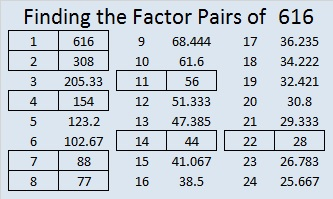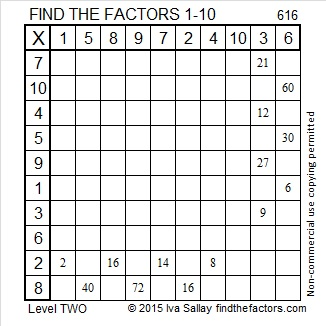# 616 and Level 2

The Padovan sequence produces a lovely spiral of equilateral triangles similar to the spiral made from golden rectangles and the Fibonacci sequence.

The Padovan sequence begins with the following numbers: 1, 1, 1, 2, 2, 3, 4, 5, 7, 9, 12, 16, 21, 28, 37, 49, 65, 86, 114, 151, 200, 265, 351, 465, 616, 816, 1081 …

The first two numbers in the Fibonacci sequence are both 1’s. After that a number, n, in the Fibonacci sequence is found by adding together (n-2) and (n-1).

The first three numbers in the Padovan sequence are all 1’s. After that a number, n, in the Padovan sequence is found by adding together (n-3) and (n-2), and 616 is one of those numbers.Print the puzzles or type the solution on this excel file: 10 Factors 2015-09-14

—————————————————————————————————

• 616 is a composite number.
• Prime factorization: 616 = 2 x 2 x 2 x 7 x 11, which can be written 616 = (2^3) x 7 x 11
• The exponents in the prime factorization are 1, 3, and 1. Adding one to each and multiplying we get (3 + 1)(1 + 1)(1 + 1) = 4 x 2 x 2 = 16. Therefore 616 has exactly 16 factors.
• Factors of 616: 1, 2, 4, 7, 8, 11, 14, 22, 28, 44, 56, 77, 88, 154, 308, 616
• Factor pairs: 616 = 1 x 616, 2 x 308, 4 x 154, 7 x 88, 8 x 77, 11 x 56, 14 x 44, or 22 x 28
• Taking the factor pair with the largest square number factor, we get √616 = (√4)(√154) = 2√154 ≈ 24.819347.—————————————————————————————————This site uses Akismet to reduce spam. Learn how your comment data is processed.